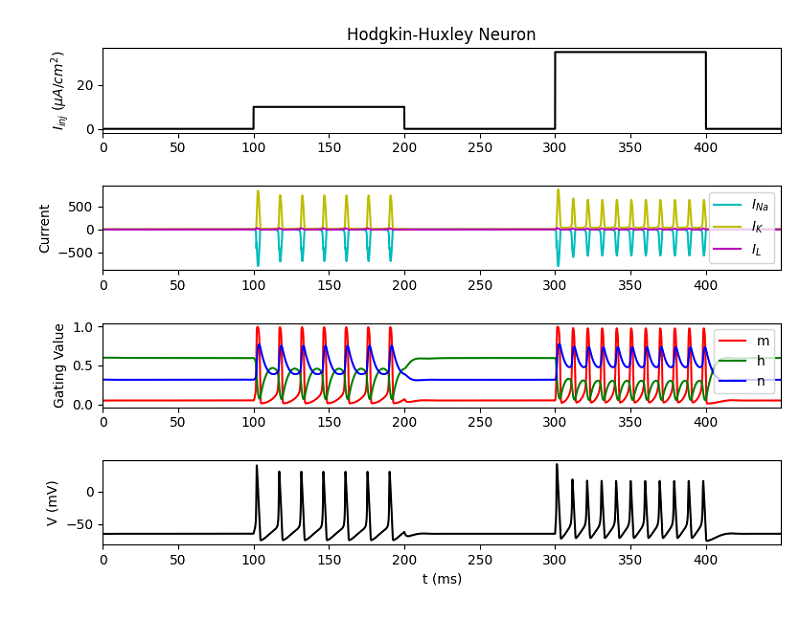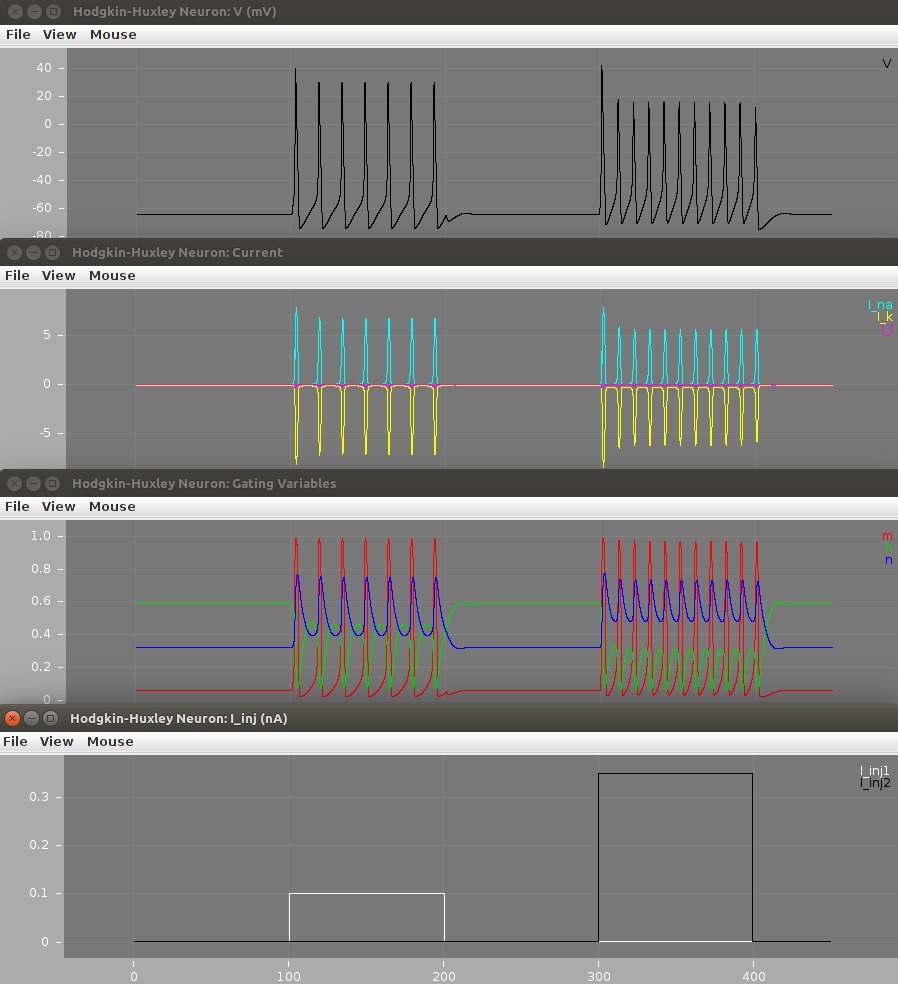# Implementation of HH Model in Python and NeuroML 2¶

In this section, we make line-by-line comparisons of the contents of the HodgkinHuxley.py python script and the contents of the NeuroML 2 files and the related LEMS_HH_Simulation.xml LEMS file.

## Installing the code¶

You can either clone a local copy of this repository from GitHub using:

```git clone https://github.com/openworm/hodgkin_huxley_tutorial.git
cd hodgkin_huxley_tutorial/Tutorial/Source
```

or just download a zip file of the latest code, extract the contents and go to hodgkin_huxley_tutorial-master/Tutorial/Source/.

## Running the model implementations¶

To run the Python version:

```python HodgkinHuxley.py
```

To run the NeuroML 2 version on Linux/Mac:

```./run.sh
```

or on Windows:

```run.bat
```

These both use the bundled jar file generated from jNeuroML. Alternatively, you can install the latest jNeuroML or pyNeuroML and use the corresponding command line utilities to run the model:

```jnml LEMS_HH_Simulation.xml
pynml LEMS_HH_Simulation.xml
```

### Membrane Capacitance¶

This variable from HodgkinHuxley.py:

```    def __init__(self, C_m=1, gmax_Na=120, gmax_K=36, gmax_L=0.3, E_Na=50,
E_K=-77, E_L=-54.387, t_0=0, t_n=450, delta_t=0.01,
I_inj_amplitude=0, I_inj_duration=0, I_inj_delay=0,
vc_delay=10, vc_duration=30, vc_condVoltage=-65,
```

Is used in this line in hhcell.cell.nml:

```                <specificCapacitance value="1.0 uF_per_cm2"/>
```

### Sodium (Na) Ion Channel Variables¶

These variables from HodgkinHuxley.py:

```    def __init__(self, C_m=1, gmax_Na=120, gmax_K=36, gmax_L=0.3, E_Na=50,
E_K=-77, E_L=-54.387, t_0=0, t_n=450, delta_t=0.01,
injected_current_plot=True, gating_plot=True, cond_scaling_plot=False,
cond_dens_plot=True, driving_force_plot=False,
```
```
self.gmax_K  = gmax_K
```

Are used in this line in hhcell.cell.nml:

```                <channelDensity id="naChans" ionChannel="naChan" condDensity="120.0 mS_per_cm2" erev="50.0 mV" ion="na"/>
```

### Potassium (K) Ion Channel Variables¶

These variables from HodgkinHuxley.py:

```    def __init__(self, C_m=1, gmax_Na=120, gmax_K=36, gmax_L=0.3, E_Na=50,
E_K=-77, E_L=-54.387, t_0=0, t_n=450, delta_t=0.01,

self.C_m  = C_m
```
```
self.gmax_L  = gmax_L
```

Are used in this line in hhcell.cell.nml:

```                <channelDensity id="kChans" ionChannel="kChan" condDensity="36 mS_per_cm2" erev="-77mV" ion="k"/>
```

### Passive Leak Channel Variables¶

These variables from HodgkinHuxley.py:

```    def __init__(self, C_m=1, gmax_Na=120, gmax_K=36, gmax_L=0.3, E_Na=50,
E_K=-77, E_L=-54.387, t_0=0, t_n=450, delta_t=0.01,

self.gmax_Na = gmax_Na
```
```
self.E_Na = E_Na
```

Are used in this line in hhcell.cell.nml:

```                <channelDensity id="leak" ionChannel="passiveChan" condDensity="0.3 mS_per_cm2" erev="-54.387mV" ion="non_specific"/>
```

### Time of Simulation¶

This variable from HodgkinHuxley.py:

```    def __init__(self, C_m=1, gmax_Na=120, gmax_K=36, gmax_L=0.3, E_Na=50,
E_K=-77, E_L=-54.387, t_0=0, t_n=450, delta_t=0.01,

self.E_K  = E_K
```

Is used in this line in LEMS_HH_Simulation.xml:

```    <Simulation id="sim1" length="450ms" step="0.01ms" target="HHCellNetwork">
```

This specifies that the simulation should run for 450 milliseconds and use a step size for integration of 0.01 milliseconds.

### Input Current / Input Current Density¶

The method from HodgkinHuxley.py takes the input in as a current density in the form of uA/cm^2. NeuroML/LEMS uses an input current in the form of nA, which requires a conversion in the input values.

This method from HodgkinHuxley.py:

 ``` 1 2 3 4 5 6 7 8 9 10 11 12 13 14 15 16 17 18``` ``` def I_inj(self, t): """ External Current | :param t: time | :return: step up to 10 uA/cm^2 at t>100 | step down to 0 uA/cm^2 at t>200 | step up to 35 uA/cm^2 at t>300 | step down to 0 uA/cm^2 at t>400 """ """ running standalone python script """ if __name__ == '__main__': return 10*(t>100) - 10*(t>200) + 35*(t>300) - 35*(t>400) #""" running jupyterLab notebook """ else: return self.I_inj_amplitude*(t>self.I_inj_delay) - self.I_inj_amplitude*(t>self.I_inj_delay+self.I_inj_duration) ```

By using a given surface area of 1000.0 um^2 in the cell, it makes the conversion from uA/cm^2 to nA easier.```            <segment id="0" name="soma">
<proximal x="0" y="0" z="0" diameter="17.841242"/> <!--Gives a convenient surface area of 1000.0 um^2-->
<distal x="0" y="0" z="0" diameter="17.841242"/>
</segment>
```

Given a surface area of 1000.0 um^2 in the cell the following equation is used to convert from X uA/cm^2 to Y nA:Line 11 can then be translated into the delay, duration and amplitude of the two pulseGenerator objects in HHCellNetwork.net.nml:

```    <network id="HHCellNetwork">
```

### Channel Gating Kinetics for Sodium (Na) Channel m¶

m is the activation variable for the Sodium (Na) Channel.

The function that governs the activation of this channel is based on the overall membrane voltage, because the channel opens and closes based on detecting the membrane potential.

These methods from HodgkinHuxley.py:

 ```1 2 3``` ``` def alpha_m(self, V): """Channel gating kinetics. Functions of membrane voltage""" return 0.1*(V+40.0)/(1.0 - np.exp(-(V+40.0) / 10.0)) ```
 ```1 2 3``` ``` def beta_m(self, V): """Channel gating kinetics. Functions of membrane voltage""" return 4.0*np.exp(-(V+65.0) / 18.0) ```

Are used in these lines in naChan.channel.nml:

```        <gateHHrates id="m" instances="3">
<forwardRate type="HHExpLinearRate" rate="1per_ms" midpoint="-40mV" scale="10mV"/>
<reverseRate type="HHExpRate" rate="4per_ms" midpoint="-65mV" scale="-18mV"/>
</gateHHrates>
```

### Channel Gating Kinetics for Sodium (Na) Channel h¶

h is the inactivation variable for the Sodium (Na) Channel. Inactivation is a different state than not being activated, which is called “deactivated”. You can read more about how Sodium channel gating works.

The function that governs the activation of this channel is based on the overall membrane voltage, because the channel opens and closes based on detecting the membrane potential.

These methods from HodgkinHuxley.py:

 ```1 2 3``` ``` def alpha_h(self, V): """Channel gating kinetics. Functions of membrane voltage""" return 0.07*np.exp(-(V+65.0) / 20.0) ```
 ```1 2 3``` ``` def beta_h(self, V): """Channel gating kinetics. Functions of membrane voltage""" return 1.0/(1.0 + np.exp(-(V+35.0) / 10.0)) ```

Are used in these lines in naChan.channel.nml:

```        <gateHHrates id="h" instances="1">
<forwardRate type="HHExpRate" rate="0.07per_ms" midpoint="-65mV" scale="-20mV"/>
<reverseRate type="HHSigmoidRate" rate="1per_ms" midpoint="-35mV" scale="10mV"/>
</gateHHrates>
```

### Channel Gating Kinetics for Potassium (K) channel n¶

n is the activation variable for the Potassium (K) Channel. The potassium channel does not inactivate, so there is no inactivation variable.

The function that governs the activation of this channel is based on the overall membrane voltage, because the channel opens and closes based on detecting the membrane potential.

These methods from HodgkinHuxley.py:

 ```1 2 3``` ``` def alpha_n(self, V): """Channel gating kinetics. Functions of membrane voltage""" return 0.01*(V+55.0)/(1.0 - np.exp(-(V+55.0) / 10.0)) ```
 ```1 2 3``` ``` def beta_n(self, V): """Channel gating kinetics. Functions of membrane voltage""" return 0.125*np.exp(-(V+65) / 80.0) ```

Are used in these lines in kChan.channel.nml:

```        <gateHHrates id="n" instances="4">
<forwardRate type="HHExpLinearRate" rate="0.1per_ms" midpoint="-55mV" scale="10mV"/>
<reverseRate type="HHExpRate" rate="0.125per_ms" midpoint="-65mV" scale="-80mV"/>
</gateHHrates>
```

### Initial Values¶

This line from HodgkinHuxley.py:

```    @staticmethod
```

Is used to define the initial values for the model in hhcell.cell.nml:

```                <initMembPotential value="-65mV"/>
```

The values for m, h, n at t=0 in LEMS/NML2 are worked out as the steady state values (inf) of each activation variable for the given initial membrane potential. See here for the NML2 implementation (see On Start).

You could refactor the script to do this too by introducing tau_m() and inf_m() and using alpha_m etc., change the expressions for dmdt etc. (e.g. dm/dt = (inf_m - m) / tau_m) etc. and:

```V_init = -65
X = odeint(self.dALLdt, [V_init, m_inf(V_init), h_inf(V_init), n_inf(V_init)], self.t, args=(self,))
```

### Plots¶

This line in HodgkinHuxley.py:

```        m = X[:,1]
h = X[:,2]
n = X[:,3]
ina = self.I_Na(V, m, h)
```

Is used in these lines in LEMS_HH_Simulation.xml:

```        <Display id="d1" title="Hodgkin-Huxley Neuron: V (mV)" timeScale="1ms" xmin="-20" xmax="470" ymin="-90" ymax="50">
<Line id="V" quantity="hhpop/v" scale="1mV" color="#000000" timeScale="1ms"/>
</Display>
```

This line in HodgkinHuxley.py:

```
self.run_mode='iclamp'

if '-iclamp' in sys.argv:     #default mode
self.run_mode='iclamp'
```

Is used in these lines in LEMS_HH_Simulation.xml:

```        <Display id="d3" title="Hodgkin-Huxley Neuron: Current" timeScale="1ms" xmin="-20" xmax="470" ymin="-10" ymax="10">
<Line id="I_na" quantity="hhpop/bioPhys1/membraneProperties/naChans/iDensity" scale="1"  color="#00ffff" timeScale="1ms"/>
<Line id="I_k" quantity="hhpop/bioPhys1/membraneProperties/kChans/iDensity" scale="1"  color="#ffff00" timeScale="1ms"/>
<Line id="I_l" quantity="hhpop/bioPhys1/membraneProperties/leak/iDensity" scale="1"  color="#ff00ff" timeScale="1ms"/>
</Display>
```

This line in HodgkinHuxley.py:

```
if self.is_vclamp():
self.t = np.arange(0, 50, 0.001)           #update default time array for python script (notebook can be controlled through widgets)

# init_values are the steady state values for v,m,h,n at zero current injection
```

Is used in these lines in LEMS_HH_Simulation.xml:

```        <Display id="d2" title="Hodgkin-Huxley Neuron: Gating Variables" timeScale="1ms" xmin="-20" xmax="470" ymin="-0.1" ymax="1.1">
<Line id="m" quantity="hhpop/bioPhys1/membraneProperties/naChans/naChan/m/q" scale="1"  color="#ff0000" timeScale="1ms"/>
<Line id="h" quantity="hhpop/bioPhys1/membraneProperties/naChans/naChan/h/q" scale="1"  color="#00dd00" timeScale="1ms"/>
<Line id="n" quantity="hhpop/bioPhys1/membraneProperties/kChans/kChan/n/q" scale="1"  color="#0000ff" timeScale="1ms"/>
</Display>
```

This line in HodgkinHuxley.py:

```        """
Main demo for the Hodgkin Huxley neuron model
```

Is used in these lines in LEMS_HH_Simulation.xml:

```        <Display id="d4" title="Hodgkin-Huxley Neuron: I_inj (nA)" timeScale="1ms" xmin="-20" xmax="470" ymin="-0.01" ymax="0.4">
<Line id="I_inj1" quantity="hhpop/pulseGen1/i" scale="1nA"  color="#ffffff" timeScale="1ms"/>
<Line id="I_inj2" quantity="hhpop/pulseGen2/i" scale="1nA"  color="#000000" timeScale="1ms"/>
</Display>
```

### Output of simulations¶

After running the scripts the output figures should look like the ones below.

For: python HodgkinHuxley.pyFor: run.sh (or run.bat on Windows)Check out the electrophysiology part of this tutorial for an explanation of these plots.